# 10kHz to 225MHz VFO/RF Generator with Si5351 - Version 2

For use in DIY homebrew radio equipment such superheterodyne single/double conversion receiver, SDR, HAM QRP transceivers or RF generator.

IntermediateWork in progress82,171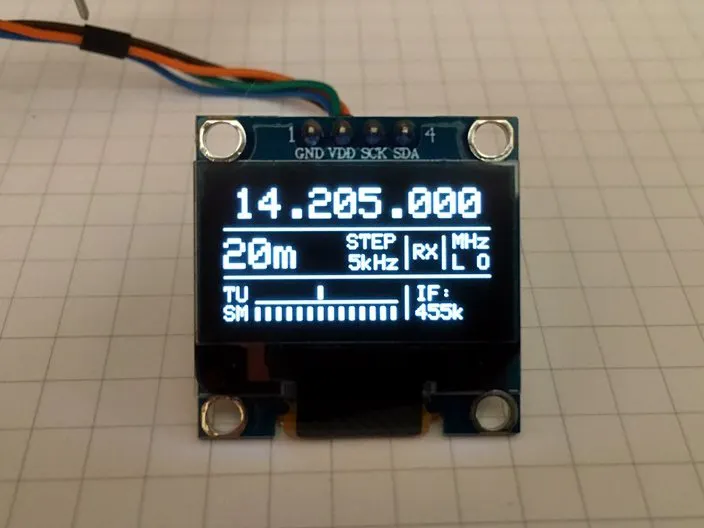## Things used in this project

### Hardware components×1Arduino Nano R3
×1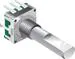Rotary Encoder with Push-Button
×1
×1
×1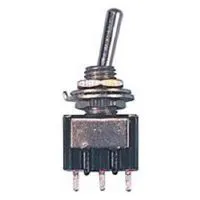Toggle Switch, SPDT
×2
 Panasonic RCA JACK FOR RF OUTPUT CONECTION
×2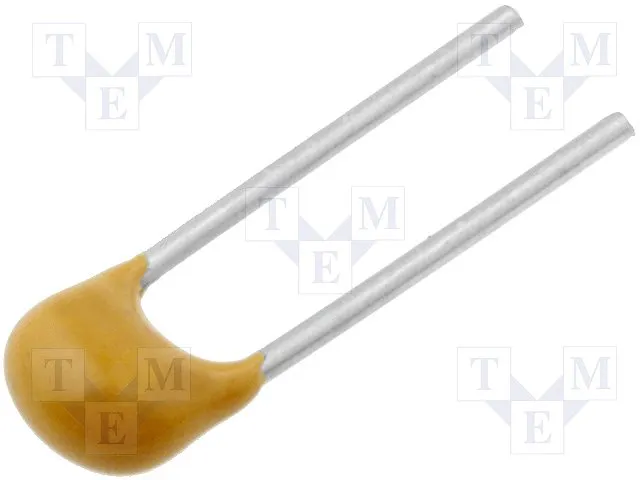Capacitor 100 nF
×3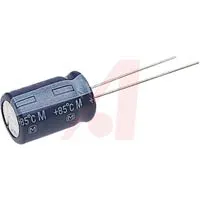Capacitor 10 µF
×1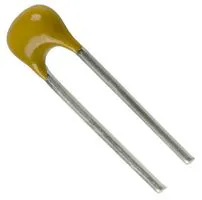Capacitor 10 nF
×2Resistor 1k ohm
×1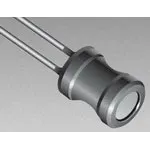Inductor 100 uH
×1

### Software apps and online servicesArduino IDE

## Schematics

### Schematics wiring

Wiring the circuit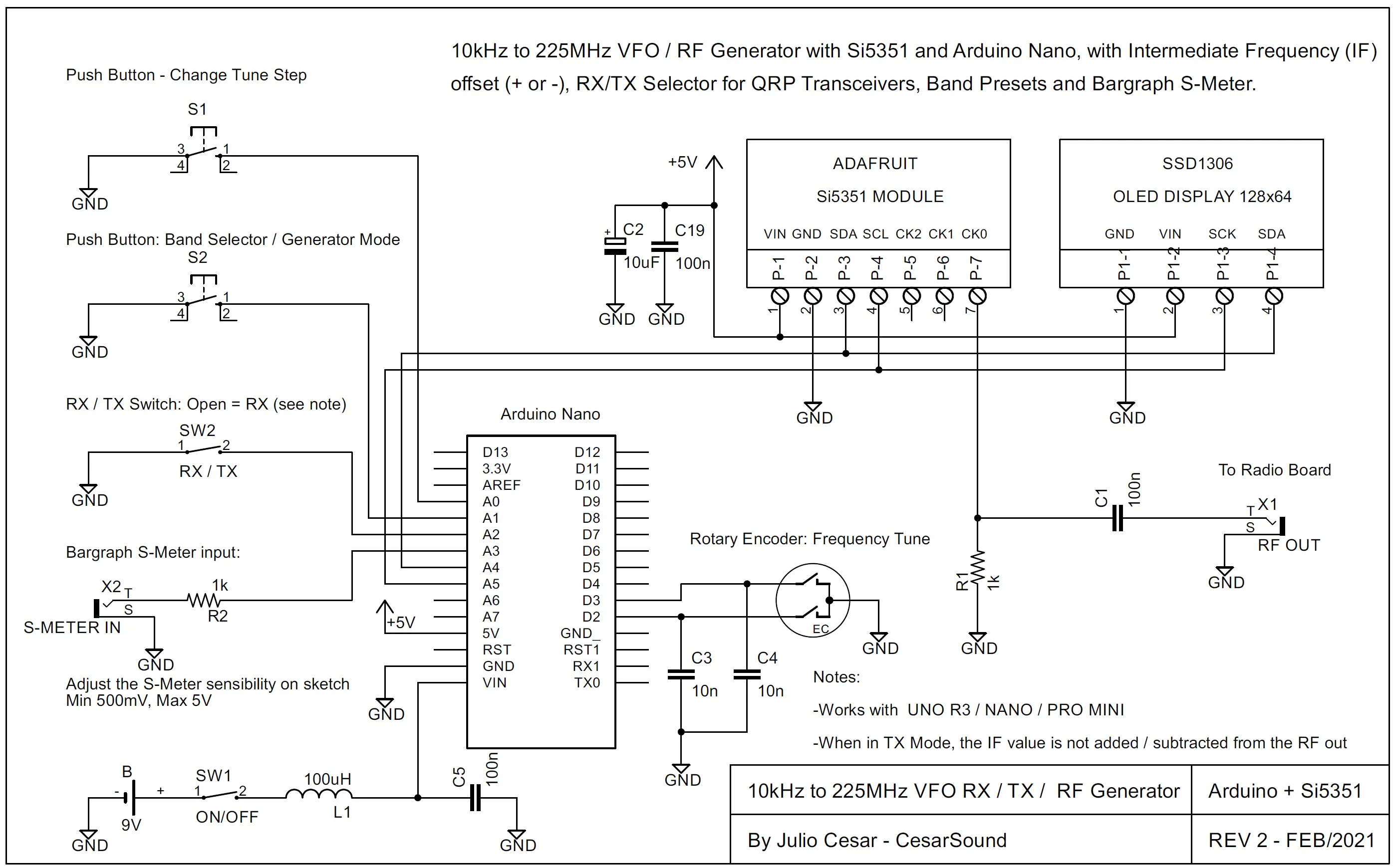## Code

### Sketch SI5351_VFO_RF_GEN_OLED_JCR_V2

C/C++
```/**********************************************************************************************************
10kHz to 225MHz VFO / RF Generator with Si5351 and Arduino Nano, with Intermediate Frequency (IF) offset
(+ or -), RX/TX Selector for QRP Transceivers, Band Presets and Bargraph S-Meter. See the schematics for
wiring and README.txt for details. By J. CesarSound - ver 2.0 - Feb/2021.
***********************************************************************************************************/

//Libraries
#include <Wire.h>                 //IDE Standard
#include <Rotary.h>               //Ben Buxton https://github.com/brianlow/Rotary
#include <si5351.h>               //Etherkit https://github.com/etherkit/Si5351Arduino

//User preferences
//------------------------------------------------------------------------------------------------------------
#define IF         455       //Enter your IF frequency, ex: 455 = 455kHz, 10700 = 10.7MHz, 0 = to direct convert receiver or RF generator, + will add and - will subtract IF offfset.
#define BAND_INIT  7         //Enter your initial Band (1-21) at startup, ex: 1 = Freq Generator, 2 = 800kHz (MW), 7 = 7.2MHz (40m), 11 = 14.1MHz (20m).
#define XT_CAL_F   33000     //Si5351 calibration factor, adjust to get exatcly 10MHz. Increasing this value will decreases the frequency and vice versa.
#define S_GAIN     303       //Adjust the sensitivity of Signal Meter A/D input: 101 = 500mv; 202 = 1v; 303 = 1.5v; 404 = 2v; 505 = 2.5v; 1010 = 5v (max).
#define tunestep   A0        //The pin used by tune step push button.
#define band       A1        //The pin used by band selector push button.
#define rx_tx      A2        //The pin used by RX / TX selector switch, RX = switch open, TX = switch closed to GND. When in TX, the IF value is not considered.
#define adc        A3        //The pin used by Signal Meter A/D input.
//------------------------------------------------------------------------------------------------------------

Rotary r = Rotary(2, 3);
Si5351 si5351(0x60); //Si5351 I2C Address 0x60

unsigned long freq, freqold, fstep;
long interfreq = IF, interfreqold = 0;
long cal = XT_CAL_F;
unsigned int smval;
byte encoder = 1;
byte stp, n = 1;
byte count, x, xo;
bool sts = 0;
unsigned int period = 100;
unsigned long time_now = 0;

ISR(PCINT2_vect) {
char result = r.process();
if (result == DIR_CW) set_frequency(1);
else if (result == DIR_CCW) set_frequency(-1);
}

void set_frequency(short dir) {
if (encoder == 1) {                         //Up/Down frequency
if (dir == 1) freq = freq + fstep;
if (freq >= 225000000) freq = 225000000;
if (dir == -1) freq = freq - fstep;
if (fstep == 1000000 && freq <= 1000000) freq = 1000000;
else if (freq < 10000) freq = 10000;
}
if (encoder == 1) {                       //Up/Down graph tune pointer
if (dir == 1) n = n + 1;
if (n > 42) n = 1;
if (dir == -1) n = n - 1;
if (n < 1) n = 42;
}
}

void setup() {
Wire.begin();
display.begin(SSD1306_SWITCHCAPVCC, 0x3C);
display.clearDisplay();
display.setTextColor(WHITE);
display.display();

pinMode(2, INPUT_PULLUP);
pinMode(3, INPUT_PULLUP);
pinMode(tunestep, INPUT_PULLUP);
pinMode(band, INPUT_PULLUP);
pinMode(rx_tx, INPUT_PULLUP);

//statup_text();  //If you hang on startup, comment

si5351.set_correction(cal, SI5351_PLL_INPUT_XO);
si5351.drive_strength(SI5351_CLK0, SI5351_DRIVE_8MA);
si5351.output_enable(SI5351_CLK0, 1);                  //1 - Enable / 0 - Disable CLK
si5351.output_enable(SI5351_CLK1, 0);
si5351.output_enable(SI5351_CLK2, 0);

PCICR |= (1 << PCIE2);
PCMSK2 |= (1 << PCINT18) | (1 << PCINT19);
sei();

count = BAND_INIT;
bandpresets();
stp = 4;
setstep();
}

void loop() {
if (freqold != freq) {
time_now = millis();
tunegen();
freqold = freq;
}

if (interfreqold != interfreq) {
time_now = millis();
tunegen();
interfreqold = interfreq;
}

if (xo != x) {
time_now = millis();
xo = x;
}

time_now = (millis() + 300);
setstep();
delay(300);
}

time_now = (millis() + 300);
inc_preset();
delay(300);
}

time_now = (millis() + 300);
sts = 1;
} else sts = 0;

if ((time_now + period) > millis()) {
displayfreq();
layout();
}
}

void tunegen() {
si5351.set_freq((freq + (interfreq * 1000ULL)) * 100ULL, SI5351_CLK0);
}

void displayfreq() {
unsigned int m = freq / 1000000;
unsigned int k = (freq % 1000000) / 1000;
unsigned int h = (freq % 1000) / 1;

display.clearDisplay();
display.setTextSize(2);

char buffer = "";
if (m < 1) {
display.setCursor(41, 1); sprintf(buffer, "%003d.%003d", k, h);
}
else if (m < 100) {
display.setCursor(5, 1); sprintf(buffer, "%2d.%003d.%003d", m, k, h);
}
else if (m >= 100) {
unsigned int h = (freq % 1000) / 10;
display.setCursor(5, 1); sprintf(buffer, "%2d.%003d.%02d", m, k, h);
}
display.print(buffer);
}

void setstep() {
switch (stp) {
case 1: stp = 2; fstep = 1; break;
case 2: stp = 3; fstep = 10; break;
case 3: stp = 4; fstep = 1000; break;
case 4: stp = 5; fstep = 5000; break;
case 5: stp = 6; fstep = 10000; break;
case 6: stp = 1; fstep = 1000000; break;
}
}

void inc_preset() {
count++;
if (count > 21) count = 1;
bandpresets();
delay(50);
}

void bandpresets() {
switch (count)  {
case 1: freq = 100000; tunegen(); break;
case 2: freq = 800000; break;
case 3: freq = 1800000; break;
case 4: freq = 3650000; break;
case 5: freq = 4985000; break;
case 6: freq = 6180000; break;
case 7: freq = 7200000; break;
case 8: freq = 10000000; break;
case 9: freq = 11780000; break;
case 10: freq = 13630000; break;
case 11: freq = 14100000; break;
case 12: freq = 15000000; break;
case 13: freq = 17655000; break;
case 14: freq = 21525000; break;
case 15: freq = 27015000; break;
case 16: freq = 28400000; break;
case 17: freq = 50000000; break;
case 18: freq = 100000000; break;
case 19: freq = 130000000; break;
case 20: freq = 144000000; break;
case 21: freq = 220000000; break;
}
si5351.pll_reset(SI5351_PLLA);
stp = 4; setstep();
}

void layout() {
display.setTextColor(WHITE);
display.drawLine(0, 20, 127, 20, WHITE);
display.drawLine(0, 43, 127, 43, WHITE);
display.drawLine(105, 24, 105, 39, WHITE);
display.drawLine(87, 24, 87, 39, WHITE);
display.drawLine(87, 48, 87, 63, WHITE);
display.drawLine(15, 55, 82, 55, WHITE);
display.setTextSize(1);
display.setCursor(59, 23);
display.print("STEP");
display.setCursor(54, 33);
if (stp == 2) display.print("  1Hz"); if (stp == 3) display.print(" 10Hz"); if (stp == 4) display.print(" 1kHz");
if (stp == 5) display.print(" 5kHz"); if (stp == 6) display.print("10kHz"); if (stp == 1) display.print(" 1MHz");
display.setTextSize(1);
display.setCursor(92, 48);
display.print("IF:");
display.setCursor(92, 57);
display.print(interfreq);
display.print("k");
display.setTextSize(1);
display.setCursor(110, 23);
if (freq < 1000000) display.print("kHz");
if (freq >= 1000000) display.print("MHz");
display.setCursor(110, 33);
if (interfreq == 0) display.print("VFO");
if (interfreq != 0) display.print("L O");
display.setCursor(91, 28);
if (!sts) display.print("RX"); if (!sts) interfreq = IF;
if (sts) display.print("TX"); if (sts) interfreq = 0;
bandlist(); drawbargraph();
display.display();
}

void bandlist() {
display.setTextSize(2);
display.setCursor(0, 25);
if (count == 1) display.print("GEN"); if (count == 2) display.print("MW"); if (count == 3) display.print("160m"); if (count == 4) display.print("80m");
if (count == 5) display.print("60m"); if (count == 6) display.print("49m"); if (count == 7) display.print("40m"); if (count == 8) display.print("31m");
if (count == 9) display.print("25m"); if (count == 10) display.print("22m"); if (count == 11) display.print("20m"); if (count == 12) display.print("19m");
if (count == 13) display.print("16m"); if (count == 14) display.print("13m"); if (count == 15) display.print("11m"); if (count == 16) display.print("10m");
if (count == 17) display.print("6m"); if (count == 18) display.print("WFM"); if (count == 19) display.print("AIR"); if (count == 20) display.print("2m");
if (count == 21) display.print("1m");
if (count == 1) interfreq = 0; else if (!sts) interfreq = IF;
}

smval = analogRead(adc); x = map(smval, 0, S_GAIN, 1, 14); if (x > 14) x = 14;
}

void drawbargraph() {
byte y = map(n, 1, 42, 1, 14);
display.setTextSize(1);

//Pointer
display.setCursor(0, 48); display.print("TU");
switch (y) {
case 1: display.fillRect(15, 48, 2, 6, WHITE); break;
case 2: display.fillRect(20, 48, 2, 6, WHITE); break;
case 3: display.fillRect(25, 48, 2, 6, WHITE); break;
case 4: display.fillRect(30, 48, 2, 6, WHITE); break;
case 5: display.fillRect(35, 48, 2, 6, WHITE); break;
case 6: display.fillRect(40, 48, 2, 6, WHITE); break;
case 7: display.fillRect(45, 48, 2, 6, WHITE); break;
case 8: display.fillRect(50, 48, 2, 6, WHITE); break;
case 9: display.fillRect(55, 48, 2, 6, WHITE); break;
case 10: display.fillRect(60, 48, 2, 6, WHITE); break;
case 11: display.fillRect(65, 48, 2, 6, WHITE); break;
case 12: display.fillRect(70, 48, 2, 6, WHITE); break;
case 13: display.fillRect(75, 48, 2, 6, WHITE); break;
case 14: display.fillRect(80, 48, 2, 6, WHITE); break;
}

//Bargraph
display.setCursor(0, 57); display.print("SM");
switch (x) {
case 14: display.fillRect(80, 58, 2, 6, WHITE);
case 13: display.fillRect(75, 58, 2, 6, WHITE);
case 12: display.fillRect(70, 58, 2, 6, WHITE);
case 11: display.fillRect(65, 58, 2, 6, WHITE);
case 10: display.fillRect(60, 58, 2, 6, WHITE);
case 9: display.fillRect(55, 58, 2, 6, WHITE);
case 8: display.fillRect(50, 58, 2, 6, WHITE);
case 7: display.fillRect(45, 58, 2, 6, WHITE);
case 6: display.fillRect(40, 58, 2, 6, WHITE);
case 5: display.fillRect(35, 58, 2, 6, WHITE);
case 4: display.fillRect(30, 58, 2, 6, WHITE);
case 3: display.fillRect(25, 58, 2, 6, WHITE);
case 2: display.fillRect(20, 58, 2, 6, WHITE);
case 1: display.fillRect(15, 58, 2, 6, WHITE);
}
}

void statup_text() {
display.setTextSize(1); display.setCursor(13, 18);
display.print("Si5351 VFO/RF GEN");
display.setCursor(6, 40);